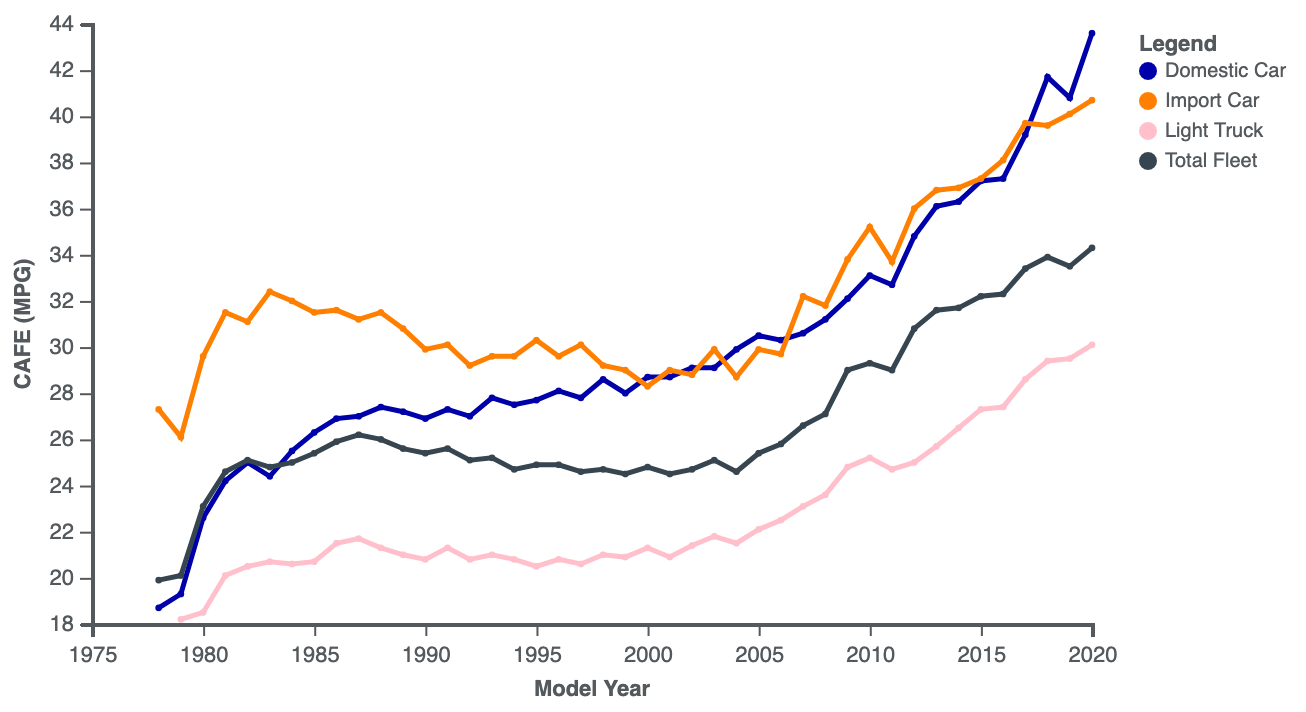# Calculator: kWh to liters through price

## Conversion from kWh/100km to Liters/100km through fuel price

There is a scientific method for converting from kWh/100 km to litres/100 km based on the energy equivalent value of fuel, but there is another good way to compare the consumption of an electric vehicle with the consumption of a petrol-fueled internal combustion engine vehicle: through the market price of fuel.

Example:

The average consumption of Tesla Model Y according to our observations is 18-21 kWh. The cost of 1 kWh in the Netherlands is 35 cents. At the same time, the cost of a liter of gasoline is 185 cents. Enter these values in the form below.

kWh / 100km = 20

Price per kWh = 35

Price per liter of gasoline = 185

We get the result: 3.78 (liters / 100 km)

Prices can be specified in any currency, it does not matter for calculation.

Enter the energy consumption value of the electric car in kWh per 100 km, the price of electricity, and the price of fuel:

The lower the energy consumption value, the more efficient your vehicle. Use the ARBA Auto app to monitor the energy efficiency of your vehicle.

Calculation algorithm:

To calculate how much gasoline can be purchased for the amount for which electricity (kWh) is purchased, you need to find out the cost of this electricity and then determine how many liters of gasoline can be purchased for this money.

1. Cost of electricity: kWh * price for 1 kWh
2. The number of liters of gasoline that can be purchased for this money: cost of electricity / price per liter

Thus, we can find out the equivalent of the energy consumed for a 100 km trip in liters of gasoline.

In the chart below, the average fuel consumption values in equivalent liters of gasoline / 100 km are shown for electric vehicles, hybrids, and internal combustion engine vehicles using various types of fuel.